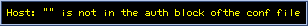## Evangelion Genesis y:x

Dec. 3, 1996. \$B\$+\$i?t\$(\$F(B\$B?ML\\$N\$*5RMM\$G\$9!#(B
\$B%a!<%k(B\$BD:\$1\$k\$H\$&\$l\$7\$\$\$G\$9!#(B

Last Updated

 \$B%_%5%H\$,E_7n\$K8F\$S=P\$5\$l\$k\$H\$3\$m\$+\$i\$=\$l\$O;O\$^\$C\$?!#(B \$BAH\$_>e\$2\$i\$l\$?IqBf\$N>e\$GMY\$k?M!9\$N9T\$-Ce\$/@h\$O(B?
\$BBh0l>O(B \$B?4\$NCV\$->l=j(B
1 \$BOC(B, 2 \$BOC(B, 3 \$BOC(B, 4 \$BOC(B, 5 \$BOC(B, 6 \$BOC(B, 7 \$BOC(B, 8 \$BOC(B, 9 \$BOC(B, 10 \$BOC(B
\$BBhFs>O(B \$B\$=\$N?H\$KGXIi\$&\$b\$N(B
11 \$BOC(B, 12 \$BOC(B, 13 \$BOC(B, 14 \$BOC(B, 15 \$BOC(B, 16 \$BOC(B, 17 \$BOC(B, 18 \$BOC(B, 19 \$BOC(B, 20 \$BOC(B, 21 \$BOC(B, 22 \$BOC(B
\$BBh;0>O(B \$BOB2r\$HBPN)\$N8~\$3\$&B&(B
y:23 \$BOD\$a\$i\$l\$7\$b\$N(B "I shall never forget."
y:24 \$BM#!"Jl?F\$H\$7\$F(B "Mother is the first other."(1/15)
\$B0J2

### y:2.x \$B%7%j!<%:(B [y:x \$B30EA%^%C%W(B]

 \$B\$4\$/F|>oE*\$J!"\$4\$/:3:Y\$JkZ\$\$\$H?(\$l9g\$\$\$NF|!9!#(By:x 2 \$BOCD>A0\$NJ*8l(B
\$BBh0l>O(B \$B88A[E*\$J\$k;W\$\$=P(B
\$BBhFs>O(B \$B5n\$j\$f\$/F|!9!"\$-\$?\$kF|!9(B
y:2.15 14 \$BG/A0!"\$3\$NF|\$K(B "The Reason why I celebrate" (12/4)

### y:x \$B30EA(B [y:x \$B30EA%^%C%W(B]

y:x.x \$B\$=\$l\$>\$l\$,;W\$&\$3\$H(B
y:5.1 \$B0U;W(B "Pray for you." (12/31)
y:24.1 \$B?4;D\$j(B "Unexperienced"(1/19)
y:25.x \$B\$A\$g\$C\$H\$7\$?;mE*>p7J(B
\$BCk2<\$,\$j(B, \$BLkL@\$1(B, \$BLsB+(B,
\$B8@\$\$Lu(B, \$B:9\$7F~\$l(B, \$B:n\$j\$9\$.(B,
\$BM)Nn\$N\$"\$kIw7J(B, \$BL25\$\$N\$"\$k8w7J(B, \$BDK\$_\$N\$"\$k>p7J(B

### \$B\$=\$NB>\$N:nIJ(B

The First Anniversary Specials
\$B?73X4|%(%t%!%s%2%j%*%s(B \$B&K(B:x
1 \$BOC(B, 2 \$BOC(B, 3 \$BOC(B, 4 \$BOC(B
EoE \$B\$K;j\$k>.JT(B
u:1 \$BL@F|(B "TOMMOROW"(12/15)
EoE \$B\$+\$i\$N>.JT(B
e:1 \$B\$=\$b\$=\$b\$N;O\$^\$j(B "In the beginning"
e:2 \$B%U%!!<%9%H(B "The First Children"
e:3 \$B\$3\$l\$+\$i(B "INITIATION"
e:0 \$B;~\$N4V7d(B "GAP"(11/1)
Orchestra Project as a Requiem
1 \$BOC(B, 2 \$BOC(B, 3 \$BOC(B, 4 \$BOC(B, 5 \$BOC(B, 6 \$BOC(B
\$B=,:n(B
\$B!V\$3\$I\$b\$N\$*\$b\$A\$c!WBh(B 66 \$BOC\$+\$i(B
\$B!V%;%s%A%a%s%?%k%8%c!<%K!(5/8)

### \$BF|;o4XO"(B

\$B:G6a\$N99?7F|;o(B(\$BB?J,FsF|\$K0l2s\$/\$i\$\$\$G99?7(B)
\$B@h7n\$N99?7F|;o(B(11 \$B7nJ,(B)
\$B0JA0\$N99?7F|;o(B
\$BF|;o>e\$N(B EVA \$B>.@b0l8@46A[\$X\$NL\
\$BF|;o>e\$N!XBg1?F02q!Y\$N46A[\$X\$NL\
\$BF|;o>e\$N(B \$B!X;R6!\$N\$*\$b\$A\$c!Y\$N46A[\$X\$NL\
\$BF|;o>e\$N(B \$B!X%;%s%A%a%s%?%k%8%c!<%K!
\$B;qNA!"\$=\$NB>(B
\$B%(%t%!>.@b%j%s%/=8(B(6/14)
\$B%a!<%k%U%)!<%`(B
\$B%j%s%/\$K\$D\$\$\$F(B

[\$B\$[!<\$`\$Z!<\$8(B] [SS \$B\$N\$Z!<\$8(B] [\$B%(%t%!\$N\$Z!<\$8(B]
\$BCi9p!";XE&!"J86g!"46A[!"\$=\$NB>\$N%a!<%k\$O\$3\$A\$i(B(kensyu@y.email.ne.jp)\$B\$^\$G(B